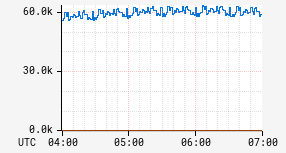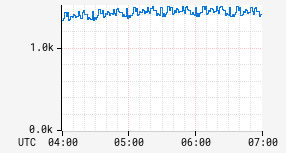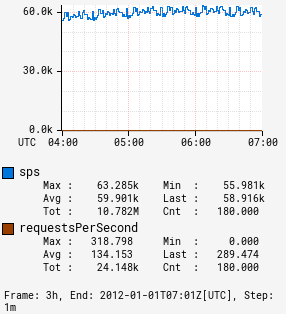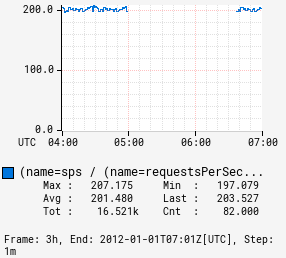# div

Input Stack:
 ts2: TimeSeriesExpr ts1: TimeSeriesExpr
Output Stack:
 (ts1 / ts2): TimeSeriesExpr

Compute a new time series where each interval has the value `(a / b)` where `a` and `b` are the corresponding intervals in the input time series. If `a` and `b` are 0, then 0 will be returned for the interval. If only `b` is 0, then NaN will be returned as the value for the interval. Sample data:

:div 0.5 0.0 NaN NaN NaN
Input 1 1.0 0.0 1.0 1.0 NaN
Input 2 2.0 0.0 0.0 NaN NaN

Use the fdiv operator to get strict floating point behavior.

Example dividing a constant:

 Before After```name,sps,:eq, 42 ``` ```name,sps,:eq, 42,:div ```

Example adding two series:

 Before After```name,sps,:eq, :sum, name,requestsPerSecond,:eq, :max, (,name,),:by ``` ```name,sps,:eq, :sum, name,requestsPerSecond,:eq, :max, (,name,),:by, :div ```